Check Important Questions and Chapter Summary -https://you.tube/Real-Numbers-Class-10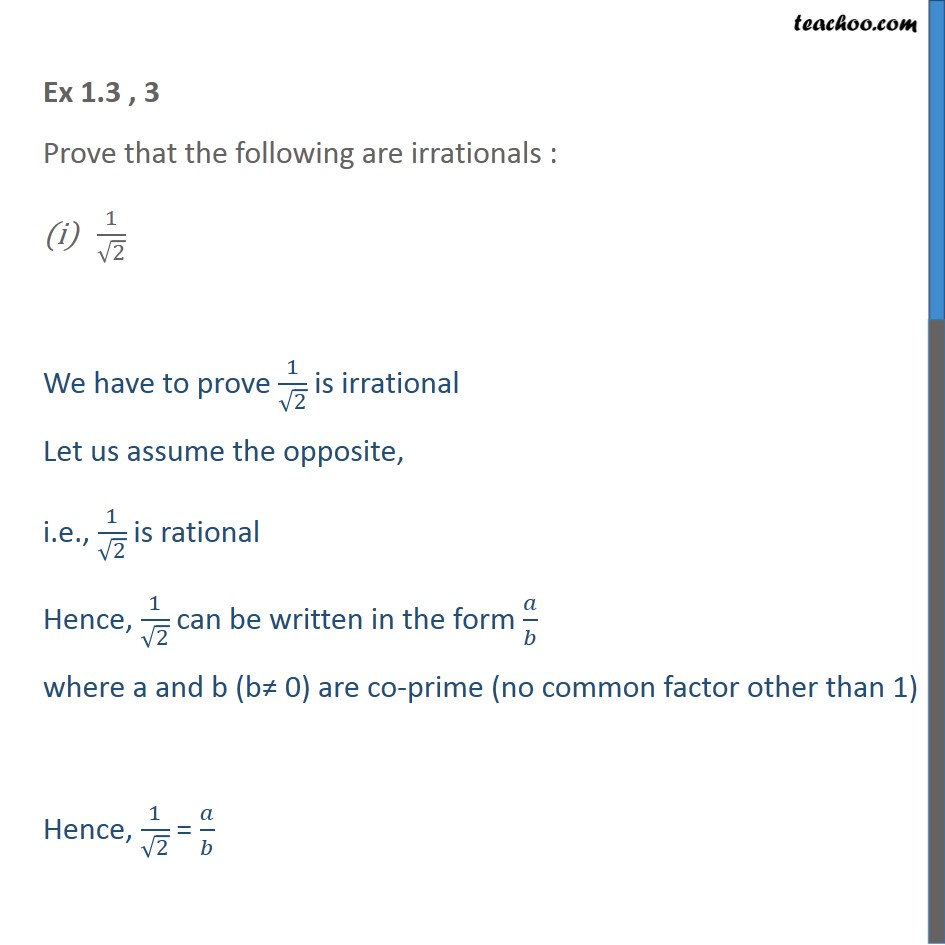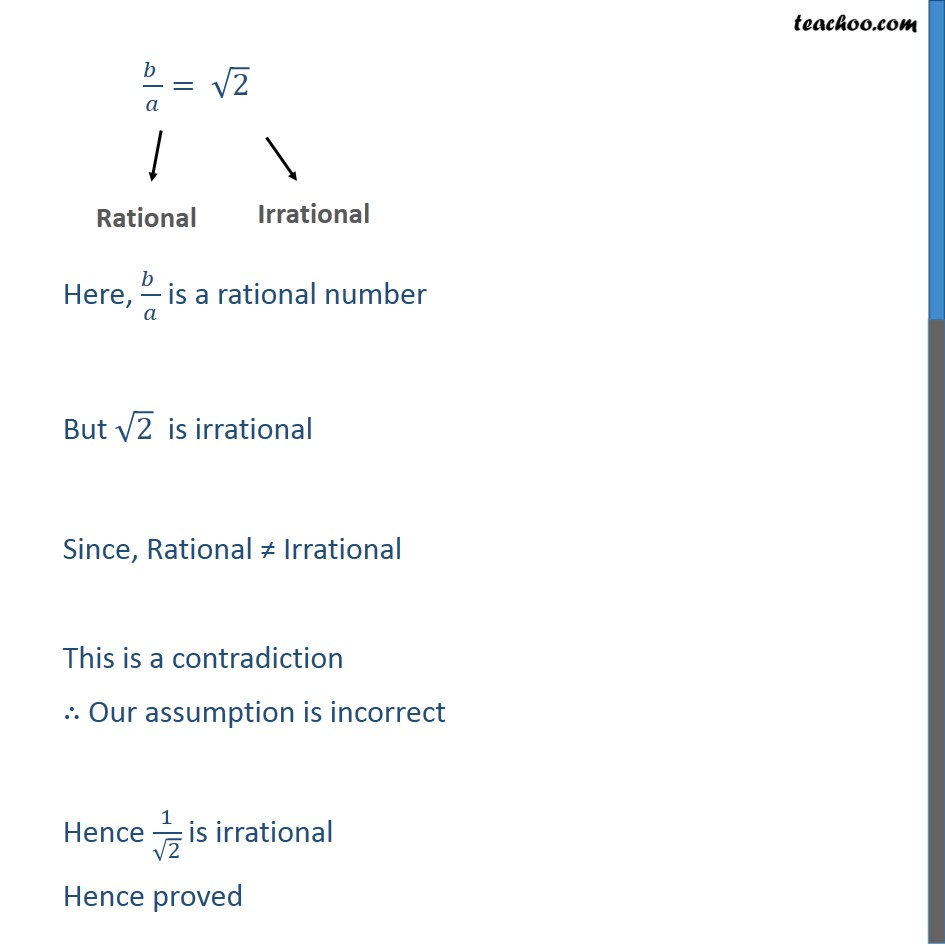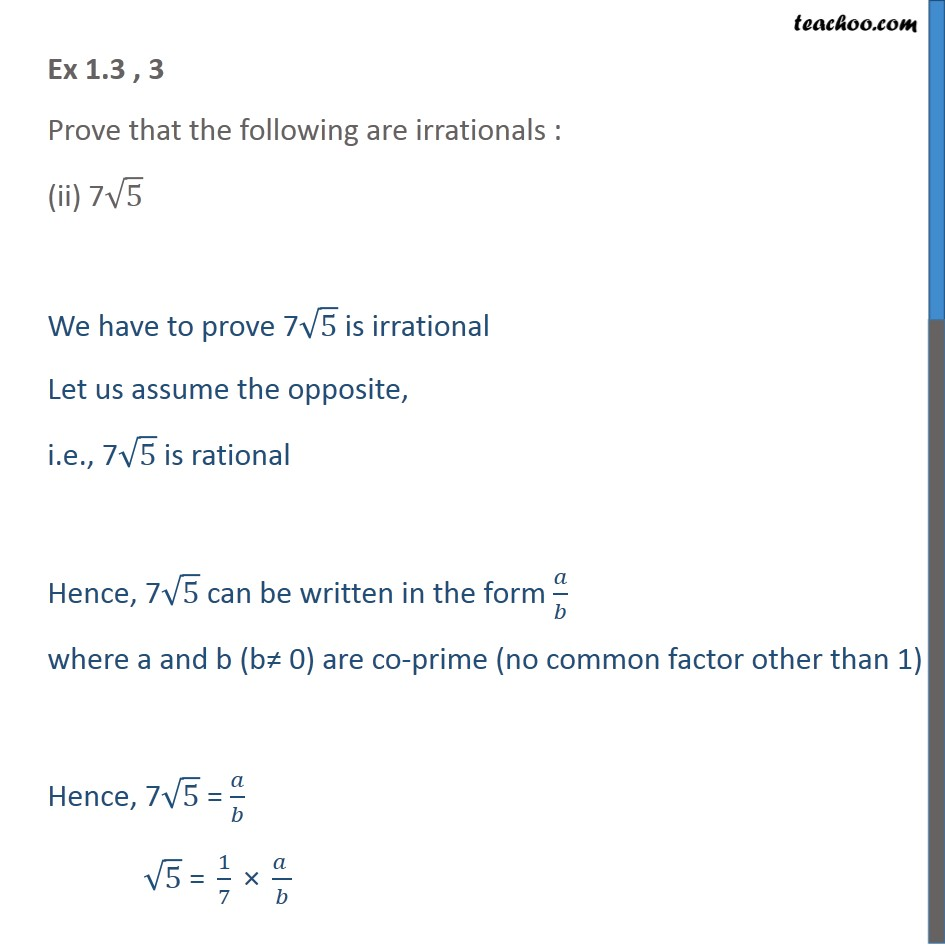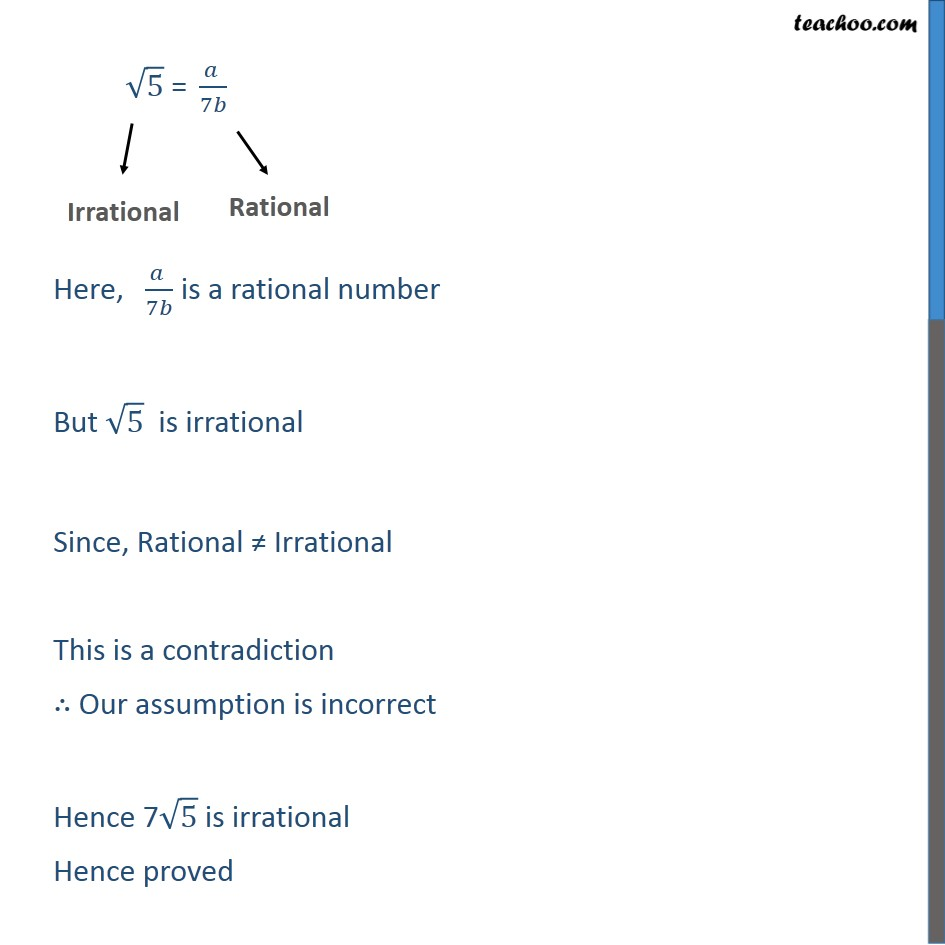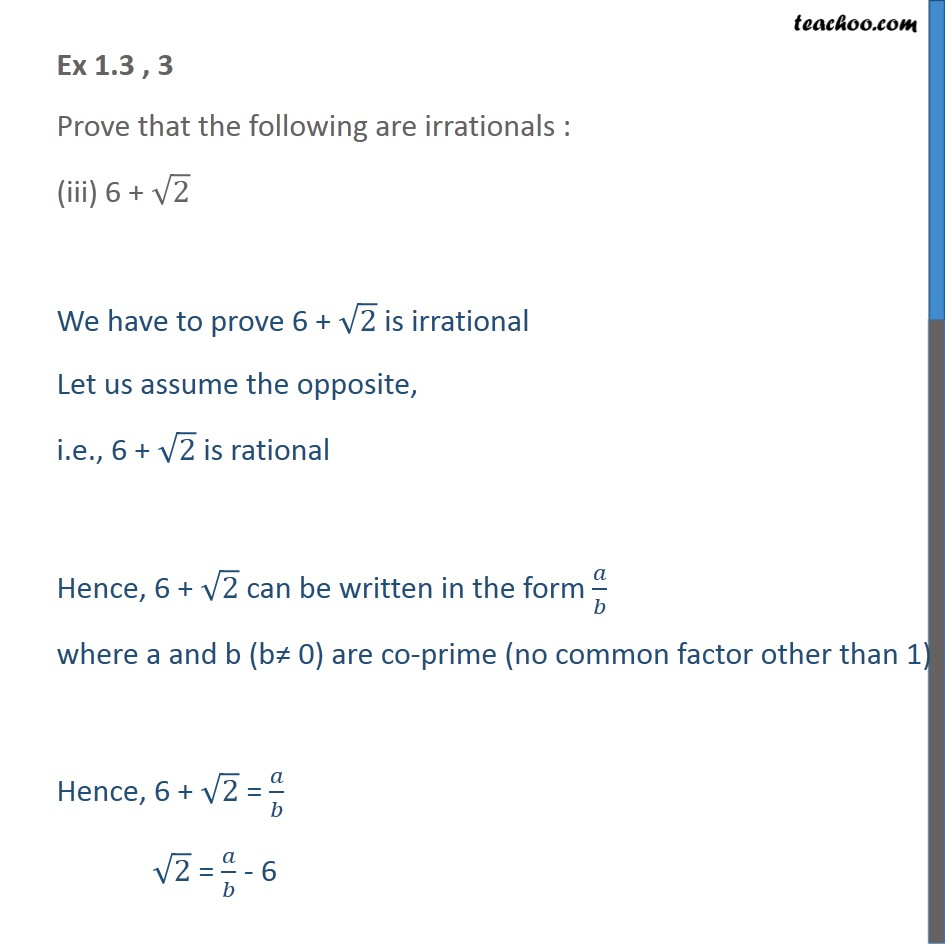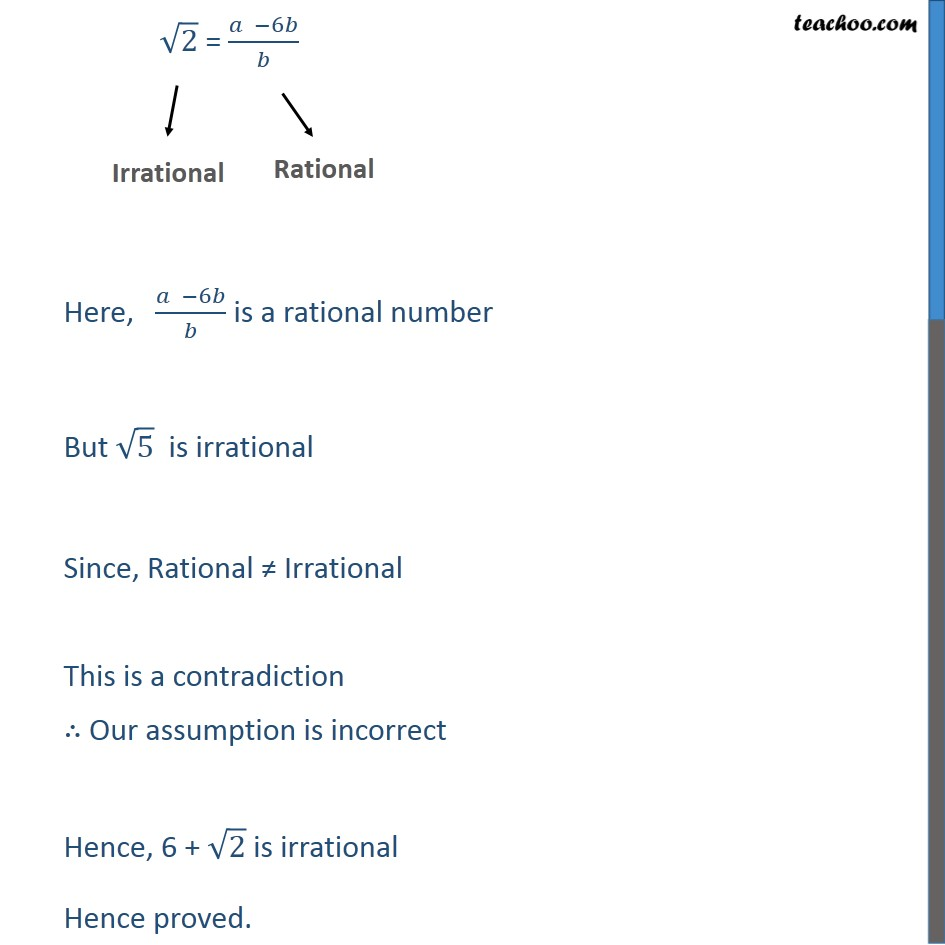1. Chapter 1 Class 10 Real Numbers
2. Concept wise
3. Irrational numbers

Transcript

Ex 1.3 , 3 Prove that the following are irrationals : 1/√2 We have to prove 1/√2 is irrational Let us assume the opposite, i.e., 1/√2 is rational Hence, 1/√2 can be written in the form 𝑎/𝑏 where a and b (b≠ 0) are co-prime (no common factor other than 1) Hence, 1/√2 = 𝑎/𝑏 (𝑏 )/𝑎= √2 " " Here, (𝑏 )/𝑎 is a rational number But √2 is irrational Since, Rational ≠ Irrational This is a contradiction ∴ Our assumption is incorrect Hence 1/√2 is irrational Hence proved Ex 1.3 , 3 Prove that the following are irrationals : (ii) 7√5 We have to prove 7√5 is irrational Let us assume the opposite, i.e., 7√5 is rational Hence, 7√5 can be written in the form 𝑎/𝑏 where a and b (b≠ 0) are co-prime (no common factor other than 1) Hence, 7√5 = 𝑎/𝑏 √5 " = " 1/7 " × " (𝑎 )/𝑏 " " √5 " = " (𝑎 )/7𝑏 Here, (𝑎 )/7𝑏 is a rational number But √5 is irrational Since, Rational ≠ Irrational This is a contradiction ∴ Our assumption is incorrect Hence 7√5 is irrational Hence proved Ex 1.3 , 3 Prove that the following are irrationals : (iii) 6 + √2 We have to prove 6 + √2 is irrational Let us assume the opposite, i.e., 6 + √2 is rational Hence, 6 + √2 can be written in the form 𝑎/𝑏 where a and b (b≠ 0) are co-prime (no common factor other than 1) Hence, 6 + √2 = 𝑎/𝑏 √2 = 𝑎/𝑏 - 6 √2 = (𝑎 −6𝑏)/𝑏 Here, (𝑎 −6𝑏)/𝑏 is a rational number But √5 is irrational Since, Rational ≠ Irrational This is a contradiction ∴ Our assumption is incorrect Hence, 6 + √2 is irrational Hence proved.

Irrational numbers